# Lie group, solvable

A Lie group that is solvable as an abstract group (cf. Solvable group). In what follows real or complex solvable Lie groups are considered.

A nilpotent, in particular an Abelian, Lie group is solvable. Ifis a complete flag in a finite-dimensional vector space(overor), thenis a solvable algebraic subgroup ofand, in particular, a solvable Lie group. If one chooses a basis incompatible with the flag, then in it the elements of the group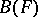are represented by non-singular upper triangular matrices; the resulting solvable matrix Lie group is denoted by, where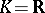or.

The Lie algebraof the groupis solvable if and only if the connected component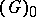of the identity ofis solvable. The Lie algebras of the groupsand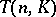areand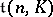, respectively (see Lie algebra, solvable). By virtue of the correspondence between subalgebras ofand connected Lie subgroups of, all properties of solvable Lie algebras carry over to solvable Lie groups (see , ).

An analogue of Lie's theorem on solvable Lie algebras is true for solvable Lie groups: Ifis a finite-dimensional complex representation of a solvable Lie group, then there is a complete flaginsuch that. In particular, inthere is a common eigen vector for all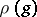,.

Solvable Lie groups were first considered by S. Lie, who conjectured that continuous groups could play the same role in the theory of integration of differential equations by quadratures as Galois groups do in the theory of algebraic equations. However, generally speaking, the group of automorphisms of a differential equation is trivial, and so meaningful results in this direction have been obtained only for linear and some other equations. Thus, for these equations the fact that the solutions can be expressed by quadratures and exponentials of them is actually equivalent to the fact that the corresponding (matrix) Galois group is solvable . If this group is nilpotent, then exponentials of quadratures do not occur in the solution.

By the Levi–Mal'tsev theorem on the decomposition of an arbitrary simply-connected Lie group into a semi-direct product (cf. Levi–Mal'tsev decomposition), solvable Lie groups play an important role in the study of arbitrary Lie groups. In an arbitrary connected Lie groupone also considers maximal solvable subgroups. If, they are Borel subgroups (cf. also Borel subgroup) and are conjugate in. For example,is a Borel subgroup of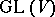.

A simply-connected solvable Lie group always has a faithful finite-dimensional representation, but for non-simply-connected solvable Lie groups this is not always so. An arbitrary connected subgroup of a simply-connected solvable Lie group is closed and simply connected . The exponential mapping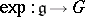need not be injective or surjective, even for a simply-connected solvable Lie group. Solvable Lie groups for which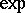is a diffeomorphism are said to be exponential (see Lie group, exponential). A simply-connected solvable Lie group is diffeomorphic to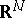, and an arbitrary connected solvable Lie group is diffeomorphic to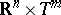, whereis an-dimensional torus.

A connected linear solvable Lie group overcan be represented as a semi-direct product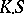, whereis a compact Abelian subgroup andis a simply-connected normal subgroup. An algebraic connected solvable group over any field of characteristic 0 splits into the semi-direct product of the normal subgroup consisting of unipotent elements and the Abelian subgroup consisting of semi-simple elements . For connected solvable Lie groups one can define  an analogue of the Mal'tsev decomposition.

If the Lie algebra of a connected Lie groupis triangular (over), thenis said to be triangular (cf. also Lie algebra, supersolvable). The analogue of Lie's theorem on solvable algebras is true for triangular Lie groups (cf. Lie theorem). Maximal connected triangular subgroups of an arbitrary connected Lie group are conjugate . A connected triangular Lie group is isomorphic to a subgroup ofand is an exponential group if it is simply connected.